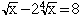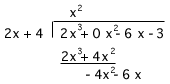/x - 2 ^4/x = 8 / = square root sign ^ = 4th root of x also, dividing 2x3 -3 -6x __________ 4 + 2x the 2x3 is 2x to the third please email as soon as possible, final week i'm studying Hi, If the answer to the first problem is an integer then I should be able to find it using guess and check. Even if the answer is not an integer, guess and check should give me an approximation to the correct answer. In the expressionthe right side is a power of 2, that is 8 = 2 3 so my intuition tells me that x will be a power of 2. Since I need to take the 4th root of x I would start with a guess of x = 2 4. If that doesn't work try x = 2 8 and then x = 2 12. For the second problem write the numerator in the form 2x 3 + 0 x 2 - 6x - 3 and proceed using long division.Can you finish it? Penny Go to Math Central